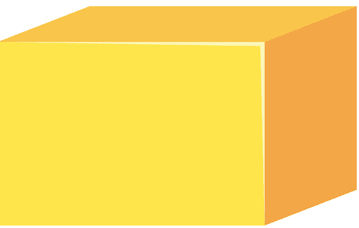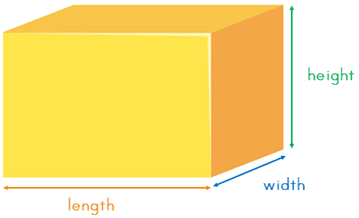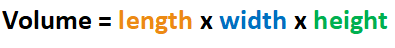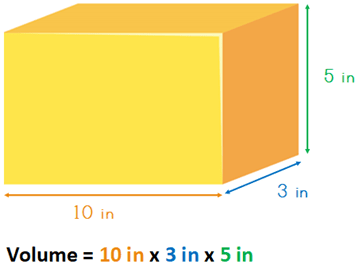The K5 Learning Blog urges parents to be pro-active in helping their children reach their full academic potential.

K5 Learning
provides free worksheets, workbooks and an online reading and math program for kindergarten to grade 5 students.# How to Measure the Volume of a Cuboid

## What is a cuboid?

A cuboid, also referred to as a rectangular prism, is a solid three-dimensional box (or object) that has six faces that are all rectangles. Here’s an example of a cuboid:

##What is volume?

The volume is the amount the three-dimensional shape takes up.

## How do we measure the volume of the cuboid?

Let’s say the cuboid is empty. We want to find out how much liquid the cuboid can contain – the volume of that shape.

If you look at this shape, there are three dimensions and, therefore, three different measurements: length, width and height. In order to work out the volume we need to multiply these three measurements.The formula for the volume of a cuboid is:Sometimes we shorten this to:

V = lwh

Let’s use an example.The answer is: Volume = 150 in3
The volume of this cuboid is 150 cubic inches.

If you want some practice, we have worksheets on finding the volume of cuboids in our grade 4 geometry section.